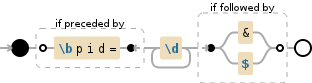# Need regex for the pattern in URL [closed]

I am writing a web crawler for some specific needs and need to tell whether a particular pattern is present in a string or not.

Supose the url which is in `String` format is like below:

``````/life-is-what-you-make-it/dask23442knk24?pid=9788129124913&ref=9090912adqeqeqeqeq
``````

If `pid` contains `only digits` (here you can see `pid=9788129124913` so it should return true for this) than do something. Can somebody help me in getting the regex for that. Thanks.

EDIT

I have been doing this by `substring()` but its too tedious so i thought of `regex` but could not comprehend it to write it by myself. Very soon i will look into regex stuff after this long pending stuff finishes. Thanks for bearing with me.

• Have you tried anything ? – Konstantin Yovkov Dec 1 '13 at 12:25
• @downvoter please at least put a comment. – Trying Dec 1 '13 at 16:02

Here is a regex:

``````\bpid=\d+(&|\$)
``````

spelled: After a word boundary, "pid=" followed by some digits, then either an ampersand or the end of the string.

(Be sure to use Matcher.find, not Matcher.matches)

• @kocko Why would it not work? – Ingo Dec 1 '13 at 12:48
• Sorry, my bad. I thoght the question is different. – Konstantin Yovkov Dec 1 '13 at 12:49
• What if pid is the last parameter and therefore not followed by an ampersand? – n.st Dec 1 '13 at 13:15
• Yes, @n.st, then it doesn't work. – Ingo Dec 1 '13 at 13:17
• Fixed it. (The edit hasn't been approved yet, though.) – n.st Dec 1 '13 at 13:22

To get just numeric value of the pid parameter, use regex pattern `(?<=\bpid=)\d+(?=&|\$)`However in Java you have to double each escape character `\`, so the pattern is

``````(?<=\\bpid=)\\d+(?=&|\$)
``````

``````Pattern p = Pattern.compile("(?<=\\bpid=)\\d+(?=&|\$)");
Matcher m = p.matcher(s);
if (m.find()) {
System.out.println(m.group(0));
}
``````

Test this demo code here.

If you don't care about validating the rest of the URL, and only want to match/grab the `pid` value, you can use:

`\?pid=(\d+)`

This also returns the `pid` value in the first group.

• This will return a false positive for any pid that starts with at least one digit, but contains some letters after that. – n.st Dec 1 '13 at 13:24